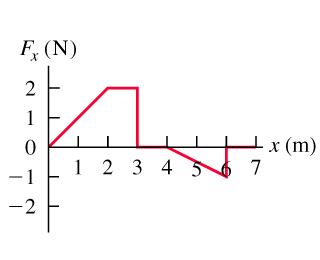# Problem: A force F is applied to a 2.0-kg radio-controlled model car parallel to the x-axis as it moves along a straight track. The x-component of the force varies with the x-coordinate of the car as shown in the figure .Calculate the work done by the force F when the car moves from x=0 to x=3.0 m.Calculate the work done by the force F when the car moves from x=3.0 m to x=4.0 m.Calculate the work done by the force F when the car moves from x=4.0 m to x=7.0 m.Calculate the work done by the force F when the car moves from x=0 to x=7.0 m.Calculate the work done by the force F when the car moves from x=7.0 m to x=2.0 m.

80% (80 ratings)
###### Problem Details

A force F is applied to a 2.0-kg radio-controlled model car parallel to the x-axis as it moves along a straight track. The x-component of the force varies with the x-coordinate of the car as shown in the figure.

Calculate the work done by the force F when the car moves from x=0 to x=3.0 m.

Calculate the work done by the force F when the car moves from x=3.0 m to x=4.0 m.

Calculate the work done by the force F when the car moves from x=4.0 m to x=7.0 m.

Calculate the work done by the force F when the car moves from x=0 to x=7.0 m.

Calculate the work done by the force F when the car moves from x=7.0 m to x=2.0 m.